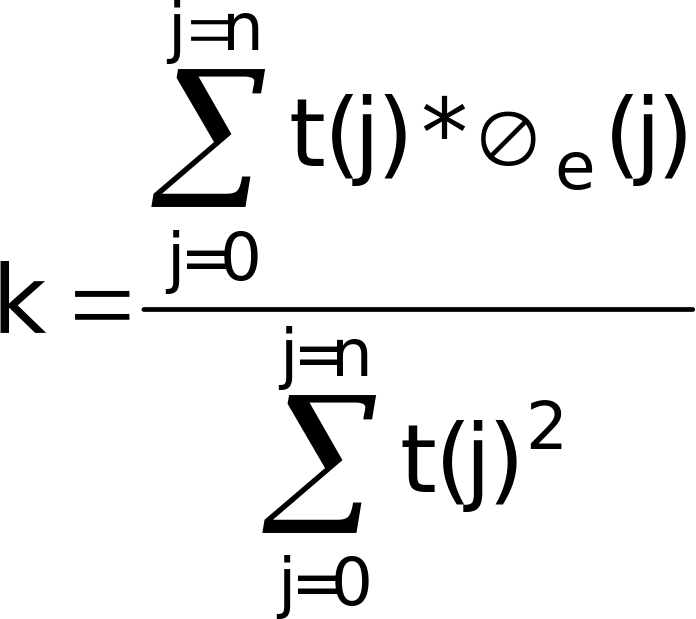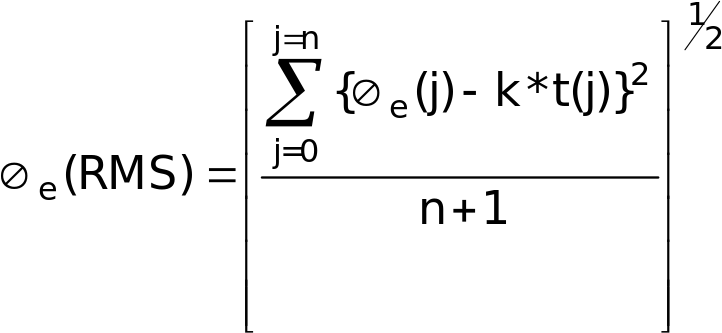## 13.17.1b Frequency error and Modulation accuracy in EC-GSM-IoT Configuration

3GPP51.010-1Mobile Station (MS) conformance specificationPart 1: Conformance specificationTS

13.17.1b.1 Definition

The frequency error is the difference in frequency, after adjustment for the effect of the modulation and phase error, between the RF transmission from the MS and either:

– the RF transmission from the BS; or

– the nominal frequency for the ARFCN used.

The phase error is the difference in phase, after adjustment for the effect of the frequency error, between the RF transmission from the MS and the theoretical transmission according to the intended modulation.

13.17.1b.2 Conformance requirement

1. The MS carrier frequency shall be accurate to within 0,1 ppm compared to signals received from the BS. For EC-GSM-IoT, the conditions shall be met at the input signal level and at the interference ratio of EC-SCH at reference performance, as defined in 3GPP TS 45.005.

1.1 Under normal conditions; 3GPP TS 45.10, subclause 6.1.

1.2 Under vibration conditions; 3GPP TS 45.10, subclause 6.1; 3GPP TS 45.05, annex D subclause D.2.3.

1.3 Under extreme conditions; 3GPP TS 45.10, subclause 6.1; 3GPP TS 45.05, subclause 4.4; 3GPP TS 45.05, annex D subclauses D.2.1 and D.2.2.

2. The RMS phase error (difference between the phase error trajectory and its linear regression on the active part of the time slot) for each burst shall not be greater than 5 degrees.

2.1 Under normal conditions; 3GPP TS 45.05, subclause 4.6.

2.2 Under vibration conditions; 3GPP TS 45.05, subclause 4.6; 3GPP TS 45.05, annex D D.2.3.

2.3 Under extreme conditions; 3GPP TS 45.05, subclause 4.6; 3GPP TS 45.05, annex D D.2.1, D.2.2.

3. The maximum peak deviation during the useful part of each burst shall not be greater than 20 degrees.

3.1 Under normal conditions; 3GPP TS 45.05, subclause 4.6.

3.2 Under vibration conditions; 3GPP TS 45.05, subclause 4.6; 3GPP TS 45.05, annex D subclause D.2.3.

3.3 Under extreme conditions; 3GPP TS 45.05, subclause 4.6; 3GPP TS 45.05, annex D subclauses D.2.1 and D.2.2.

13.17.1b.3 Test purpose

1. To verify that in a multislot configuration the MS carrier frequency error does not exceed 0.1 ppm:

1.1 Under normal conditions.

1.2 Under extreme conditions.

2. To verify that the RMS phase error on the useful parts of the bursts transmitted by the MS in a multislot configuration does not exceed conformance requirement 2:

2.1 Under normal conditions.

2.2 When the MS is being vibrated.

2.3 Under extreme conditions.

3. To verify that the maximum phase error on the useful parts of the bursts transmitted by the MS in a multislot configuration does not exceed conformance requirement 3:

3.1 Under normal conditions.

3.2 When the MS is being vibrated.

3.3 Under extreme conditions.

13.17.1b.4 Method of the test

NOTE: In order to measure the accuracy of the frequency and phase error a sampled measurement of the transmitted phase trajectory is obtained. This is compared with the theoretically expected phase trajectory. The regression line of the difference between the expected trajectory and the measured trajectory is an indication of the frequency error (assumed constant through the burst), whilst the departure of the phase differences from this trajectory is a measure of the phase error. The peak phase error is the value furthest from the regression line and the RMS phase error is the root mean square average of the phase error of all samples.

13.17.1b.4.1 Initial conditions

The test shall be run under the use of EC-PDTCH/MCS-1/16,CC4 using Overlaid CDMA with 1 selected user of 4, with power control parameter ALPHA (α) set to 0.

The SS shall command the MS to hopping mode (for the choice of frequencies in the frequency hopping mode, see subclause 6.2 and tables 6-1 and 6-2).

NOTE: It is not necessary to test in hopping mode but is done here as a simple means of making the MS change channel, it would be sufficient to test in non hopping mode and to make sure bursts are taken from a few different channels.

The MS shall be operated with its highest number of uplink slots.

The Test Mode defined in 3GPP TS 44.014 subclause 5.4 shall be utilised.

Mode (a) transmitting pseudo-random data sequence in RLC data blocks;

For the procedure described below, the initial power value of each active timeslot shall be set to a mid-range power value.

Specific PICS statements:

– MS without vibration sensitive components (TSPC_No_Vibration_Sensitive_Components)

PIXIT Statements:

13.17.1b.4.2 Procedure

a) For one transmitted burst on the last slot of the multislot configuration, the SS captures the signal as a series of phase samples over the period of the burst. These samples are evenly distributed over the duration of the burst with a minimum sampling rate of 2/T, where T is the modulation symbol period. The received phase trajectory is then represented by this array of at least 294 samples.

b) The SS then calculates, from the known bit pattern and the formal definition of the modulator contained in 3GPP TS 45.04, the expected phase trajectory.

c) From a) and b) the phase trajectory error is calculated, and a linear regression line computed through this phase trajectory error. The slope of this regression line is the frequency error of the mobile transmitter relative to the simulator reference. The difference between the regression line and the individual sample points is the phase error of that point.

c.1) The sampled array of at least 294 phase measurements is represented by the vector:

m = m(0)…m(n)

where the number of samples in the array n+1  294.

c.2) The calculated array, at the corresponding sampling instants, is represented by the vector:

c = c(0)…c(n).

c.3) The error array is represented by the vector:

e = {m(0) – c(0)}………{m(n) – c(n)} = e(0)…e(n).

c.4) The corresponding sample numbers form a vector t = t(0)…t(n).

c.5) By regression theory the slope of the samples with respect to t is k where:c.6) The frequency error is given by k/(360 * g), where g is the sampling interval in s and all phase samples are measured in degrees.

c.7) The individual phase errors from the regression line are given by:

e(j) – k*t(j).

c.8) The RMS value e of the phase errors is given by:d) Steps a) to c) are repeated for 20 bursts, not necessarily contiguous.

e) The SS instructs the MS to its maximum power control level by setting the power control parameter ALPHA () to 0 and GAMMA_TN (CH) for each timeslot to the desired power level in the Packet Uplink Assignment message (Closed Loop Control, see 3GPP TS 45.08, clause B.2), all other conditions remaining constant. Steps a) to d) are repeated.

f) The SS instructs the MS to the minimum power control level, all other conditions remaining constant. Steps a) to d) are repeated.

g) The MS is hard mounted on a vibration table and vibrated at the frequency/amplitudes specified in annex 1, TC4.

During the vibration steps a) to f) are repeated.

NOTE 1: If the call is terminated when mounting the MS to the vibration table, it will be necessary to establish the initial conditions again before repeating steps a) to f).

h) The MS is re-positioned on the vibration table in the two orthogonal planes to the plane used in step g). For each of the orthogonal planes step g) is repeated.

i) Steps a) to f) are repeated under extreme test conditions (see annex 1, TC2.2).

NOTE 2: Steps g) and h) are skipped if TSPC_No_Vibration_Sensitive_Components is declared as Yes

13.17.1b.5.1 Frequency error

For all measured bursts, the frequency error, derived in step c.6), shall be less than 10E-7.

13.17.1b.5.2 Phase error

For all measured bursts, the RMS phase error, derived in step c.8), shall not exceed 5 degrees.

For all measured bursts, each individual phase error, derived in step c.7), shall not exceed 20 degrees.